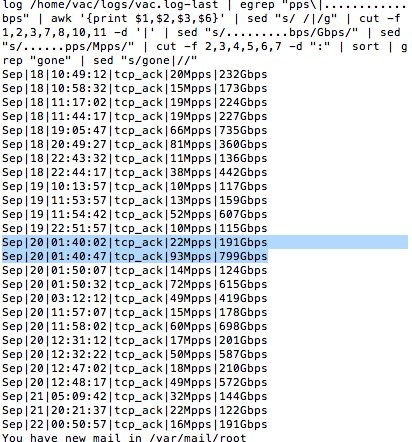# Shell - Compound Commands

A compound command is one of the following:

## Type

### (list)

````(list)`
```

where

• () defines the execution environment as a subshell
• Variable assignments and builtin commands that affect the shell’s environment do not remain in effect after the command completes.
• The return status is the exit status of list.
• list is a list

### {list}

````{ list; }`
```

where:

• {} defines a group command that is simply executed in the current shell environment.
• - Since '{}' do not cause a word break, '{}' must be separated from list by whitespace.
• list is a list
• ;: a semicolon is the terminator (or a newline)
• The return status is the exit status of list.

### ((expression))

````((expression))`
```

where:

• The expression is evaluated according to the rules described under ARITHMETIC EVALUATION.
• If the value of the expression is:
• non-zero, the return status is 0;
• otherwise the return status is 1.

This is exactly equivalent to/alias to let

````let "expression"`
```

### [[ expression ]]

Expressions may be combined using the following operators, listed in decreasing order of precedence:

### ( expression )

Returns the value of expression. This may be used to override the normal precedence of operators.

Discover MoreBash - Arithmetic Expression

arithmetic in bash part of compound expression . Counter Simple addition where let is the let command If the value of the expression is: non-zero, the return status is 0; otherwise ...Bash - Conditional Expression

A predicate or boolean expression is known in Bash as a Conditional Expression. It is a command that returns an exit status of 0 or 1. The conditional expression is part of the compound expression. ...Bash - Function

A shell function is an object that: is called like a simple command and executes a compound command with a new set of positional parameters. By convention, the function name starts with an underscore....Bash - Lists of Commands

A list of command is a sequence of one or more pipelines separated by one of the operators: ‘;’, ‘&’, ‘&&’, or ‘||’, and optionally terminated by one of ‘;’, ‘&’, or a newline....Datacadamia - Data all the things

Computer science from a data perspectiveShell - Control Operators

Symbol Pagina | & && || ; ;; ( Block definition ) Block definition